January 28, 2022

# Tutorial: Demystifying Python ListsIn this tutorial, you’ll learn how to work with Python lists as one of the most powerful data types in Python.

After you finish this tutorial, you’ll know the following:

• How to define Python lists
• How to create Python lists and access or change items within them
• How to create a list of lists
• How to slice lists
• How to add or remove items from lists
• How to use a for loop to iterate over items of a list

In this tutorial, we’re assuming that you know the fundamentals of Python, including variables, data types, and basic structures. If you’re not familiar with these or are eager to brush up your Python skills, please try our Variables, Data Types, and Lists in Python course .

## What Are Python Lists?

A Python list is a mutable collection of heterogeneous elements (items) under one variable name. In this context, mutability means the elements inside a list can be changed while the program is running. We also can add or remove elements from a list whenever we want. In Python, we define lists by enclosing the elements between square brackets and separating them with commas. Each list’s element has an index representing the element’s position in the list with a starting index of zero.

## Creating Python Lists

To define a Python list, you need to use the following syntax:

``variable_name = [item1, item2, item3, … , itemN]``

As an example, let’s create our first Python list.

``shopping_list = ["eggs", "milk", "bread", "butter"]``

The code above implements a Python list with four elements that all are strings. Now, let’s create a list that holds only the prices of what we have on the shopping list.

``price_list = [13.99, 8.50, 7.90, 12.50]``

As we mentioned in the definition of the Python lists, the list items don’t have to all be the same data type. They can be any object since everything is an object in Python.

``a_mixed_list = ["dataquest.io", 100, "python.org", 10.01, True, 'A']``

Now, let’s check the data type of these lists:

``````print(type(shopping_list))
print(type(price_list))
print(type(a_mixed_list))``````

Go ahead, and run the code. No matter which data types are stored in the lists, all the `type()` functions return `<class 'list'>`, which is the data type of list objects.

## Accessing Items Within Python Lists

As we have seen, each item in a list has its own index, so they can be retrieved using their associated indices. Let’s try the code:

``````print(shopping_list)
print(price_list)``````

Run the code; it outputs `eggs` and `7.90`. The first value is `eggs` because it is the first item in the shopping list with index 0, and the second value is `7.90` because it is the third item in the price list with index 2. The following illustration represents a list with six integer elements and their indices starting from zero. It’s also nice to know that Python supports negative indexing, which means the last element’s index is `-1`, the second to last element’s index is `-2`, and the` k`-th last element’s index is `-k`.Let’s first define the `num_list` list and then look at some examples:

``````num_list = [21, 13, 17, 39, 17, 51]
print(num_list[-1])
print(num_list)
print(num_list[-6])``````

Now run the code, and check its output. The first `print` statement returns `51`, which is the last element in the list, and the last two `print` statements return `21` because both refer to the first element of the list. Sometimes, we need to know how many elements are within a list. To do so, we use `len()` function, like this:

``print("The length of the list is {}.".format(len(num_list)))``

Running the code outputs `The length of the list is 6` because the number of elements within the list is six.

## Changing Values in Lists

Modifying an item within a list is like re-declaring a variable to a new value. Let’s look at the following example:

``````num_list = [21, 13, 17, 39, 17, 51]
print(num_list)
num_list = 33
print(num_list)``````

In the code above, before we modify the value at index 4, it outputs `[21, 13, 17, 39, 17, 51]`, and after changing the value at the index 4 to 33, it outputs` [21, 13, 17, 39, 33, 51]`. In other words, our code selects the item at position four and sets its value to 33.

## Creating a List of Lists

In Python, we can store lists within another list (nested lists). To check how we can create a nested list, let’s try the following code that makes one list containing other lists and values with different data types.

``````data = [["Python", 100], ["Java", 101], "Hello", True]
print(data)
print(data)``````

Run the code, and check its output.

``````['Python', 100], ['Java', 101], 'Hello', True]
['Java', 101]``````

The first line shows the entire list stored in the` data` variable, and the second line displays the second element of the `data` that itself is a list with two elements. So far, you’ve learned how to create nested lists. But what if we want to retrieve a specific element from an inner list. In Python, to access an item within a list, we put the item’s index between a pair of square brackets. However, if the item is inside an inner list, we have to add a second set of square brackets after the first set. Let’s check out an example:

``````print(data)
inner_list = data
print(inner_list)``````

Running the code above outputs `Python` and `Java`. In the first line of code, the first index enclosed between the first set of square brackets refers to the first item in the `data` list, which itself is a list, and the second index enclosed between the second set refers to the first value of the inner list, which results in `Python`. The illustration below represents how `Python` is retrieved from the `data` list.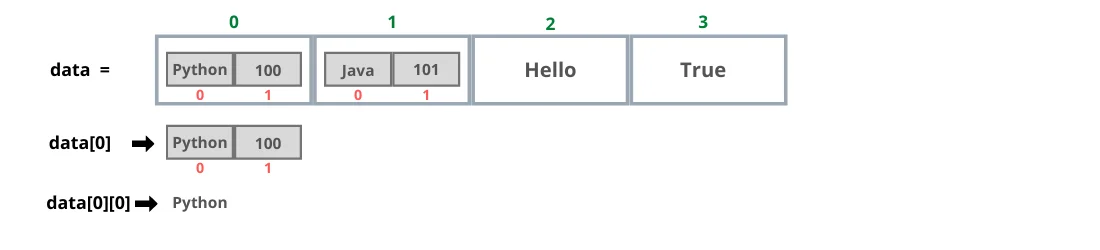In the second line of code, the value at index 1 of the `data` list, which itself is also a list assigned to the `inner_list` variable; then in the last line, we are accessing the first element of the inner list stored in the` inner_list` variable, which is `Java`. The illustration below shows how `Java` is retrieved from the `data` list.## Slicing Lists

Python allows us to create list subsets using slicing techniques. To create a list slice, we specify the index of the first and last items. The items from the first index up to, but not including, the last index are copied into the slice, and it’s evident that the copying doesn’t modify the original list. There are different ways for slicing a list; let’s look at some of them.

### Slicing with a Starting Index

Omitting the ending index means that the slice contains the list elements from the starting index to the end of the list. Let’s check it out:

``````num_list = [21, 13, 17, 39, 17, 51]
print(num_list[3:])``````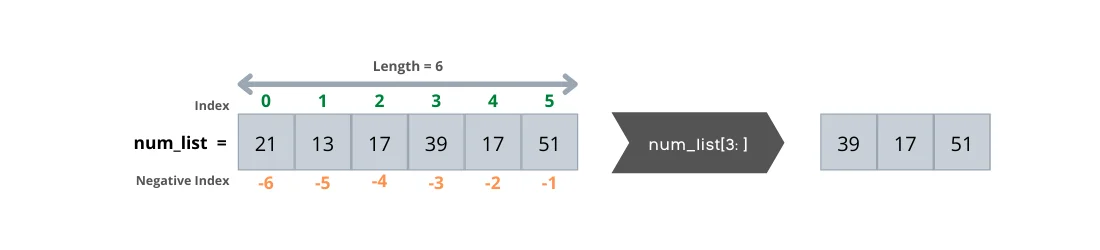### Slicing with an Ending Index

Omitting the starting index means that the slice contains the list elements from the beginning of the list up to the ending index. Let’s try the code:

``````num_list = [21, 13, 17, 39, 17, 51]
print(num_list[:4])``````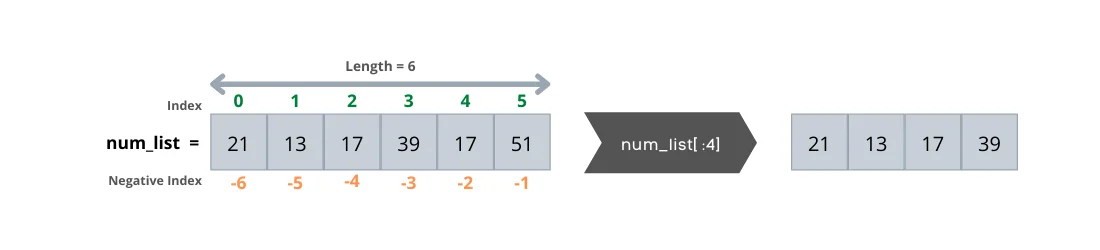NOTE

Omitting both the starting and ending indices results in the entire list.

``````print(num_list[:])
[21, 13, 17, 39, 17, 51]``````

### Slicing with Steps

We can use a step to return items at specified intervals. The step is optional — by default, it is 1. The following code returns every other item of `num_list`.

``````num_list = [21, 13, 17, 39, 17, 51]
print(num_list[ : :2])``````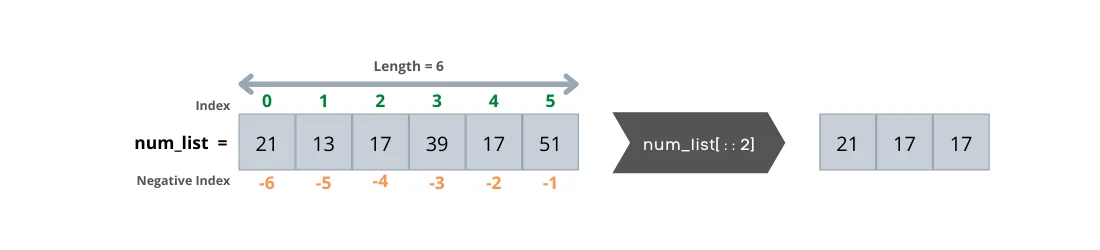### Reversing a List with Negative Step

We can reverse a list by specifying the step as -1. The following code returns the `num_list` items in reverse order.

``````num_list = [21, 13, 17, 39, 17, 51]
print(num_list[ : :-1])``````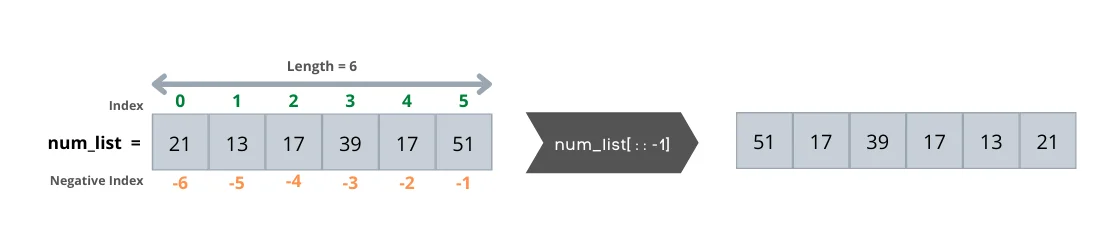NOTE

In the code above, although we didn’t specify the start and end indices, both are assumed as negative indices as follows:

``````print(num_list[-1:-9:-1])
[51, 17, 39, 17, 13, 21]``````

## Adding Items to a List

There are two methods for adding new items into a list, `insert()` and `append()` methods. The new item can be a string, a number, or even another list. We can use both methods to expand a list.

### .insert()

The` insert()` method adds an item at a specified location within a list. Let’s see how it works.

``````fruits = ["apple", "orange", "cherry"]
fruits.insert(1, "pineapple")
print(fruits)``````

The code above inserts `pineapple` into the list at index 1 and outputs as follows: `['apple', 'pineapple', 'orange', 'cherry']`

### .append()

The `append()` method adds an item at the end of a list. Let’s check it out.

``````fruits = ["apple","orange","cherry"]
fruits.append("pineapple")
print(fruits)``````

The code above adds `pineapple` at the end of the list and prints it out. `['apple', 'orange', 'cherry', 'pineapple']`

NOTE

You can add a list of objects at the end of a list with `+=` operator. If the left operand of `+=` is a list, the right operand must be a list or any other iterable object.

``````students = ["Mary", "James","Patricia"]
students += ["Robert"]
students += ["Emma", "William"]
print(students)
['Mary', 'James', 'Patricia', 'Robert', 'Emma', 'William']``````

The square brackets around the student name create a single-element list in the second line, which is appended to the `students` list using the `+=` operator. In the same way, the third line appends a list of two `students` to the students list. We also can concatenate two lists using the `+` operator. The result is a new list containing the students of class A followed by the students of class B.

``````classA = ["Mary", "James","Patricia"]
classB = ["Robert", "Emma", "William"]
students = classA + classB
print(students)
['Mary', 'James', 'Patricia', 'Robert', 'Emma', 'William']``````

## Removing Items from a List

There are two primary methods for removing items from a list, `pop()` and `remove()` methods.

### .pop()

The `pop()` method removes an item at a specified index from a list and returns the item, which can be assigned to a variable and used later. The default value of the index is -1, which means if the index value isn’t provided, it removes the last item.

``````fruits = ["apple", "orange", "cherry", "pineapple"]

second_fruit = fruits.pop(1) # removes the second item
last_fruit = fruits.pop() # removes the last item

print("Second Fruit is {}.".format(second_fruit))
print("Last Fruit is {}.".format(last_fruit))
print(fruits)``````

The code above removes two items: the first item is at index 1, and the second item is at the end of the list. Also, while popping these items out of the list, it saves them into the `second_fruit` and `last_fruit` variables, which prints them out later along with the modified `fruits` list.

``````Second Fruit is orange.
Last Fruit is pineapple.
['apple', 'cherry']``````

### .remove()

The `remove()` method removes the first occurrence of an element with a specified value.

``````fruits = ["apple", "orange", "cherry", "pineapple"]
fruits.remove('cherry')
print(fruits)``````

Running the code outputs the `fruits` list without "cherry" because the `remove()` method removed it successfully.

``['apple', 'orange', 'pineapple']``

But what happens if the item that we’re going to remove doesn’t exist in the list? Let’s run the following code and see the result:

``````fruits = ["apple", "orange", "cherry", "pineapple"]
fruits.remove('blueberry')
print(fruits)``````

The code raises an error that the value isn’t in the list. Let’s see how we can fix it by using a try-except block to catch exceptions.

``````fruits = ["apple", "orange", "cherry", "pineapple"]
try:
fruits.remove('blueberry')
print(fruits)
except:
print("The fruit name does not exist in the list.")``````

Run the code. This time our code doesn’t crash, and it outputs a proper message that the item (fruit name) doesn’t exist in the list.

``The fruit name does not exist in the list.``

### The `in` and `not in` Operators

Basically, the `in` operator checks whether a specified value is in a list or not. When the `in` operator is used in a condition, the statement returns a Boolean value evaluating into either `True` or `False`. When the specified value is found inside the list, the statement returns `True`; otherwise, it returns `False`.

``````num_list = []
if not num_list:
print(“The list is empty.”)``````

The code outputs “The list is empty” since the `num_list` is empty.

NOTE

We can create an empty list by assigning empty square brackets to a variable. The length of an empty list is zero, and we can always add elements to the empty list using the `append()` or `insert()` methods.

### The `for` Loop and Lists

We can use the `for` loop to iterate over a list of items and take one item at a time until no more items remain to process. Let’s look at the code.

``````fruits = ["apple", "orange", "cherry", "pineapple"]
for fruit in fruits:
print(fruit)``````

Running the code above prints each item within the list on a separate line. In other words, for every fruit in the `fruits` list, it prints the fruit’s name.

``````apple
orange
cherry
pineapple``````

### Conclusion

In this tutorial, you learned how to work with Python lists through simple and concise snippets. We’ll look at some advanced techniques in upcoming tutorials.